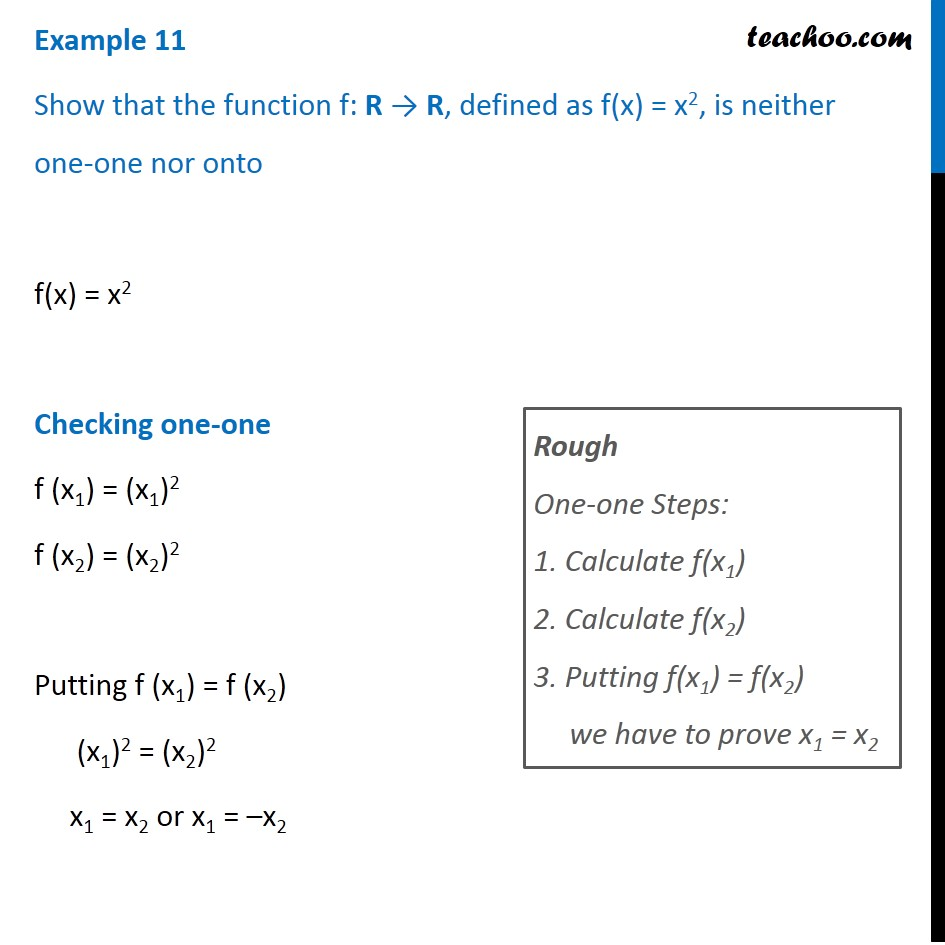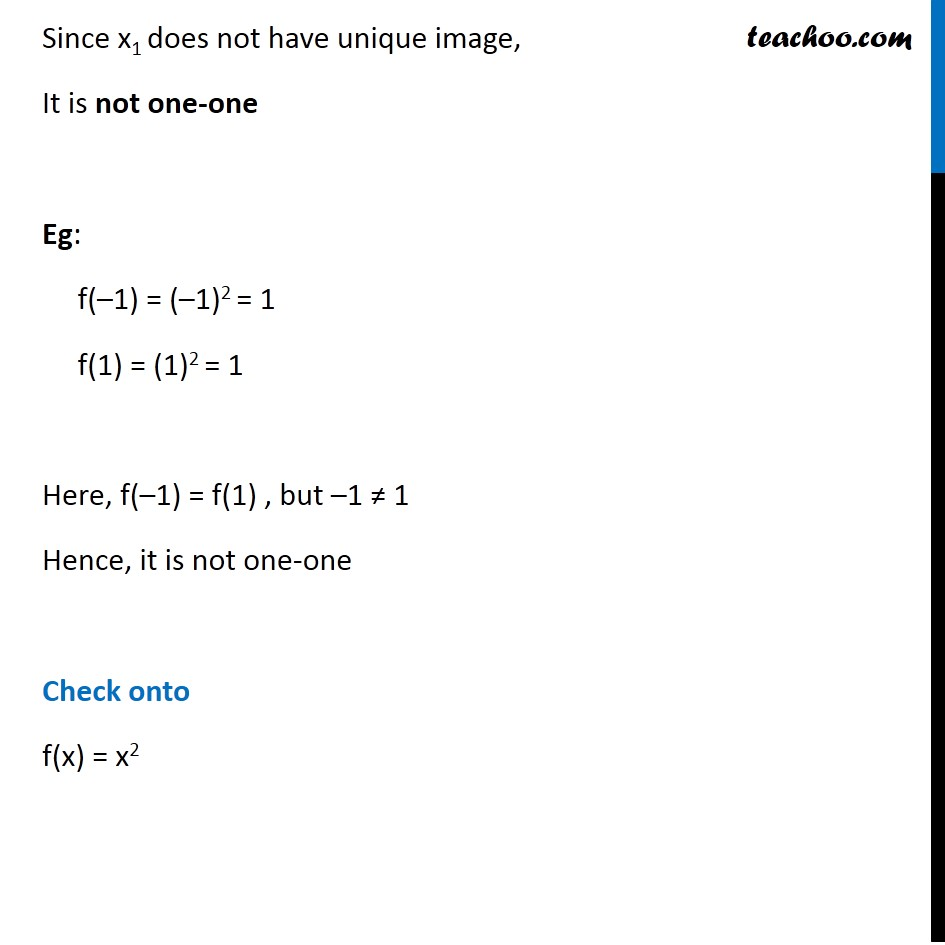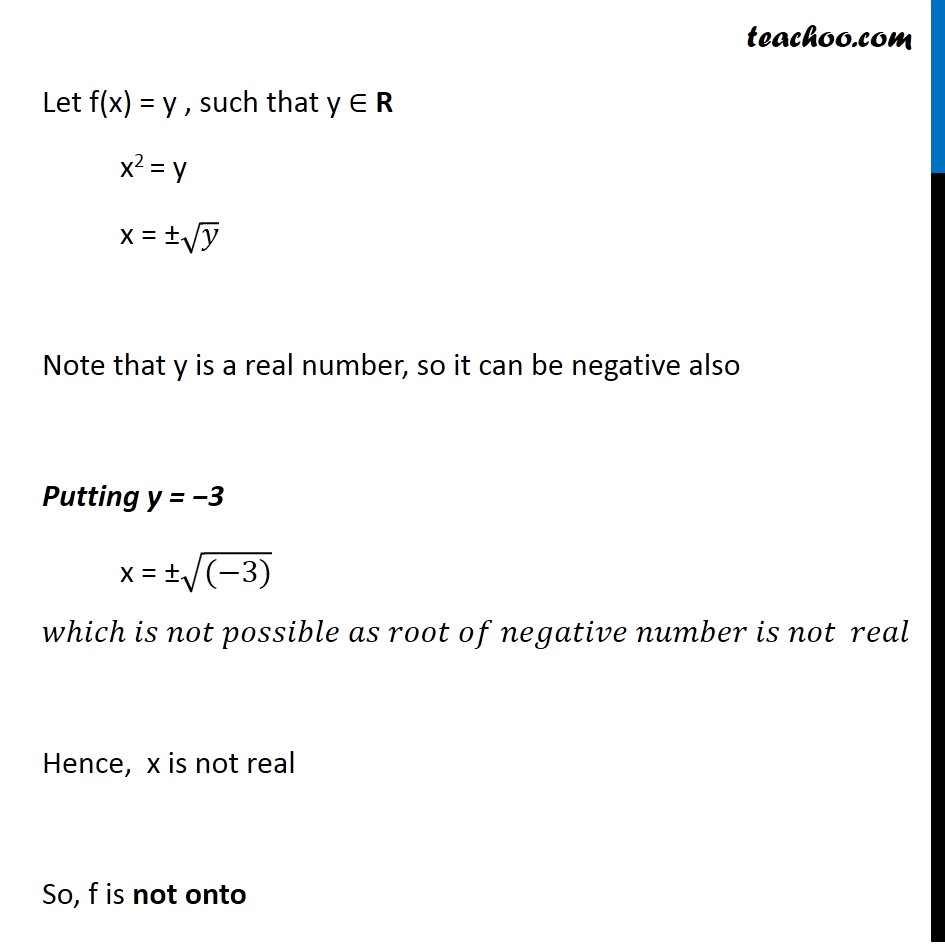Examples

Chapter 1 Class 12 Relation and Functions
Serial order wiseSolve all your doubts with Teachoo Black (new monthly pack available now!)

### Transcript

Example 11 Show that the function f: R → R, defined as f(x) = x2, is neither one-one nor onto f(x) = x2 Checking one-one f (x1) = (x1)2 f (x2) = (x2)2 Putting f (x1) = f (x2) (x1)2 = (x2)2 x1 = x2 or x1 = –x2 Rough One-one Steps: 1. Calculate f(x1) 2. Calculate f(x2) 3. Putting f(x1) = f(x2) we have to prove x1 = x2 Since x1 does not have unique image, It is not one-one Eg: f(–1) = (–1)2 = 1 f(1) = (1)2 = 1 Here, f(–1) = f(1) , but –1 ≠ 1 Hence, it is not one-one Check onto f(x) = x2 Let f(x) = y , such that y ∈ R x2 = y x = ±√𝑦 Note that y is a real number, so it can be negative also Putting y = −3 x = ±√((−3)) 𝑤ℎ𝑖𝑐ℎ 𝑖𝑠 𝑛𝑜𝑡 𝑝𝑜𝑠𝑠𝑖𝑏𝑙𝑒 𝑎𝑠 𝑟𝑜𝑜𝑡 𝑜𝑓 𝑛𝑒𝑔𝑎𝑡𝑖𝑣𝑒 𝑛𝑢𝑚𝑏𝑒𝑟 𝑖𝑠 𝑛𝑜𝑡 𝑟𝑒𝑎𝑙 Hence, x is not real So, f is not onto